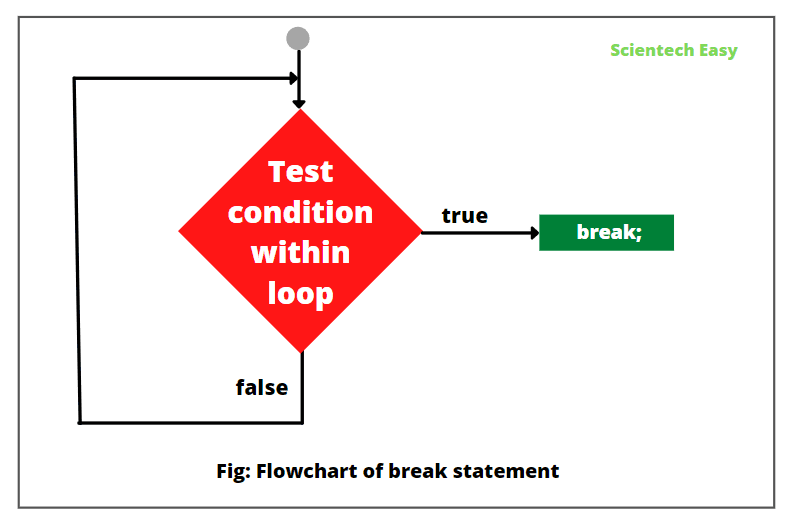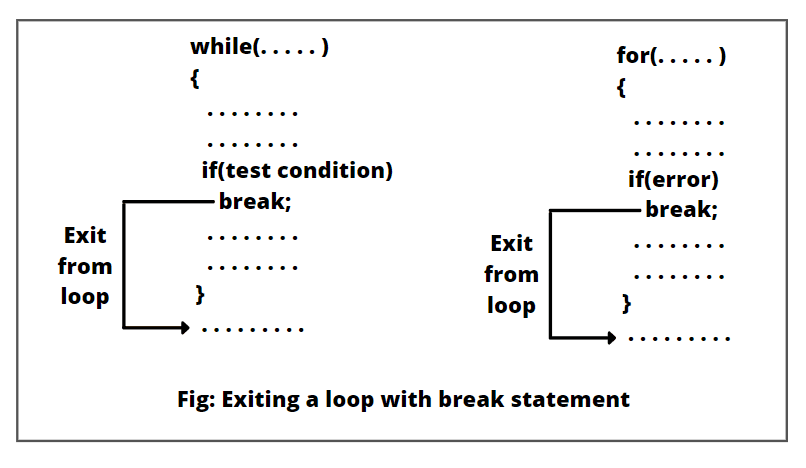# Break Statement in Java | Example Program

Java supports three types of jump statements: break, continue, and return. These statements transfer control of execution to another part of the program.

For example, suppose there is a list of 100 names and we need to search a particular name from the list. When the search completes and the desired name found, the program loop written for searching must terminate.

For this purpose, Java allows a jump from one statement to the end or beginning of a loop as well as jump out of a loop. So, let’s understand all three types of jump statements one by one.

## Break Statement in Java

A break statement in Java is used to break a loop or switch statement. When a break statement encounters inside a loop statement, the loop immediately ends at a specified condition.

As a result, the program control continues the execution of the next statement immediately following the loop body.

Similarly, when a break statement executes inside the switch block, it terminates ‘case’ inside the switch block and stops the execution of more cases inside the switch.

When the loops are nested, the break statement breaks only the inner loop. We can use Java break statement in all types of loops such as for loop, while loop, and do-while loop.

The general syntax for break statement is as follows:

```// Jump statement
break;```

The flowchart of break statement in java has shown in the below figure.You can also use the break statement with a label reference to break out of any code block. The general syntax to use break statement with label is as:

```break labelName;
```

Without a label, you can only use break inside a loop or a switch.

## Use of Break Statement

We can use a break statement in Java in three ways that are as:

1. We can use a break inside the loop to come out of it.2. We can use it inside the switch block to come out of switch block.

3. Break can also be used inside the nested blocks to go to the end of block.

## Break Statement Example Programs

1. Let’s take a simple example program where we will use a break statement inside the for loop for breaking the loop. Look at the program source code.

Program code 1:

```package javaProgram;
public class BreakExample1 {
public static void main(String[] args)
{
// Using for loop.
for(int i = 1; i <= 10; i++)
{
if(i == 5) break; // Here, the break statement is breaking a loop.
System.out.println("I: " +i);
}
}
}```
```Output:
I: 1
I: 2
I: 3
I: 4
```

2. Let’s take another example program where we will use break statement inside the inner for loop.

Program code 2:

```package javaProgram;
public class BreakExample2 {
public static void main(String[] args)
{
// Outer for loop.
for(int i = 1; i <= 3; i++)
{
// Inner for loop.
for(int j = 0; j <= 3; j++) {
if(i == 2 && j == 2)
break; // Using break statement inside for loop.
System.out.println(i+" "+j);
}
}
}
}```
```Output:
1 0
1 1
1 2
1 3
2 0
2 1
3 0
3 1
3 2
3 3
```

3. Let’s take an example program where we will use the break statement with labeled for loop. We can use break with the label. This feature was added since JDK 1.5 version.

Program code 3:

```package javaProgram;
public class BreakExample3 {
public static void main(String[] args)
{
// Outer for loop.
for(int i = 1; i <= 3; i++)
{
bb:
// Inner for loop.
for(int j = 1; j <= 3; j++){
if(i == 2 && j == 2)
break bb; // Using break statement with label.
System.out.println(i+" "+j);
}
}
}}```
```Output:
1 2
1 3
2 1
3 1
3 2
3 3
```

4. Let’s take a simple example program where we will use the break statement inside while loop.

Program code 4:

```package javaProgram;
public class BreakWhileEx {
public static void main(String[] args)
{
// while loop.
int i = 1;
while(i <= 10) {
if(i == 5) {
i++;
break; // Breaking the loop.
}
System.out.println(i);
i++;
}
}
}```
```Output:
1
2
3
4
```

5. Let’s take an example in which we will use the break statement inside the do-while loop.

Program code 5:

```package javaProgram;
public class BreakDoWhileEx {
public static void main(String[] args)
{
// Initialization.
int i = 1;

// do-while loop.
do
{
if(i == 5) {
i++;
break;
}
System.out.println(i);
i++;
} while(i <= 10);
}
}```
```Output:
1
2
3
4
```

6. Let’s take an example in which we will search an element within an array using break statement.

Program code 6:

```package javaProgram;
import java.util.Scanner;
public class SearchingEx {
public static void main(String[] args)
{
// Create a Scanner object to read user input.
Scanner scanner = new Scanner(System.in);

// Initializing a variable to keep track of the loop.
boolean found = false;

// Creating an array of five numbers.
int[] numbers = { 10, 20, 30, 40, 50 };

System.out.print("Enter a number to search for: ");
int target = scanner.nextInt();

// Using a for loop to search for the target number
for (int number : numbers)
{
if (number == target)
{
// If the target number is found, set found to true and break out of the loop
found = true;
break;
}
}

// Check if the number was found or not
if (found) {
System.out.println("Number " + target + " found in the array.");
} else {
}
// Close the Scanner object.
scanner.close();
}
}
```
```Output:
Enter a number to search for: 30
Number 30 found in the array.
```

In this example, we have taken a number as an input from the user and searches for a target number in an array. In the program, we have used the for loop to iterate through the array elements. If the target number found in the array, the loop is terminated using the break statement, and the program displays a message showing that the number was found. If the target number is not found, the loop completes naturally, and a different message is displayed.

In this tutorial, we have explained the break statement in Java with the help of different example programs. Hope that you will have understood the basic concept of using the break statement and practiced all programs. In the next, we will understand about continue statement in Java with examples.